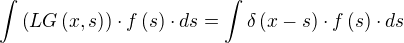## Solving of Inhomogeneous Differential Equations

Let L be a differential operator.

Say we want to solve the equationfor u

Then what we'd like to say is, that is.

However, ifhas nontrivial solutions (i.e. more than one solution: the trivial solution and another one), then L apparently cannot be injective. Hence this doesn't work.

So if L is surjective, then at least there is a way to do:(called right inverse)(the right-inverse, note that 1 is an operator)So we make the problem worse, so to speak, in order to solve for u.

One of the possible ways to construct G (which you need in order to make u worse) and (G u) is the Convolution. (G is called the Green Function)

### Convolution#### Properties of Convolution

f*g = g*f

δ*f = f*δ = f

Let the solution to the following be known:Then this G(x) is a possible Green Function.## Example

Let's say we want to solve a linear differential equation with constant coefficients (i.e. a translation invariant equation)

Let's say we have a inhomogenity f(x) acting on it:If we know a functional G so that the following holds, we can go much further:So maybe